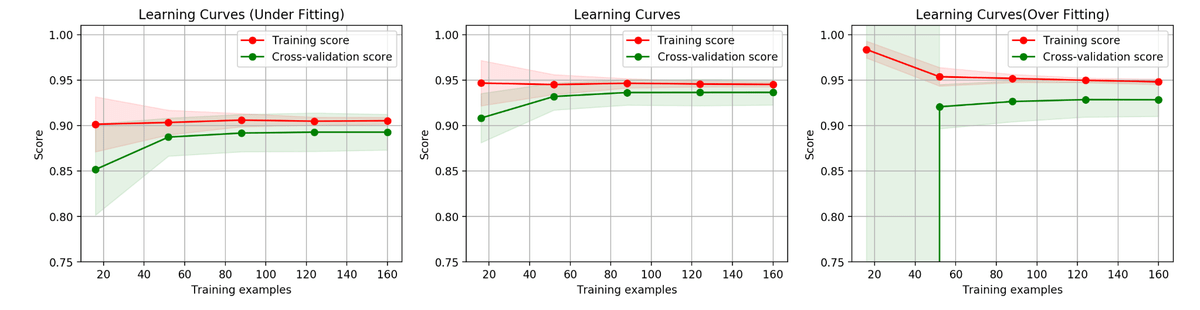# scikit-learn机器学习

## 常用算法原理及编程实战

· 机器学习,sklearn,Python

Python机器学习软件包与IPython

\$ ] pip install jupyter numpy matplotlib scipy scikit-learn seaborn

\$ ] ipython

import numpy as np
import pandas as pd
import sklearn as sk
import matplotlib as mpt

print(np.__version__)
print(pd.__version__)
print(sk.__version__)
print(mpt.__version__)

Scikit-learn

scikit-learn包含了大部分流行的监督学习算法（分类和回归）和无监督学习算法（聚类和降维）的实现。

from sklearn import datasets

images_and_labels = list(zip(digits.images, digits.target))

plt.figure(figsize=(8, 6), dpi=200)

for index, (image, label) in enumerate(images_and_labels[:8]):
plt.subplot(2, 4, index+1)
plt.axis('off')
plt.imshow(image, cmap = plt.cm.gray_r, interpolation='nearest')
plt.title('Digit:%i' % label, fontsize=20)
from sklearn.model_selection import train_test_split

#import train_test_split

Xtrain , Xtest, Ytrain, Ytest = train_test_split(digits.data, digits.target, test_size=.2, random_state=2)

from sklearn import svm

clf = svm.SVC(gamma=0.0001, C=100)
clf.fit(Xtrain, Ytrain)

sklearn所有的模型实例都有fit()接口， 用来训练模型的接口。 针对有监督学习，

fit(X, y) X是训练数据集， y是其标注。 针对监督模型， 提供predict()接口， 针对分类问题，有些模型还提供 predict_proba()接口， score接口是用来评价一个模型的好坏， 得分越高越好。

transform()接口用来进行转换， PCA算法。• more data  增大训练样本 (如果是过拟合）
• less features 减少特征，降低模型复杂(如果是过拟合）
• better feature   原有特征已经做到极致， 想要更好效果就要更好的特征输入 （如果是欠拟合）
• complex model 提升模型复杂 （如果是欠拟合）Precision = True Positive / [True Positive + False Positive]

Recall = True Positive / [True Positive + False Negative]

True Positive 表示机器准确预测出了有恶性肿瘤的观测（这里恶性 = 正样本）。

False Positive表示错误预测出了是恶性，但实际为良性。 因此Precision 即为 预测了100例， 但实际只有70例正确， 表示查准率。

sklearn.metrics.precision_score()

sklearn.metrics.recall_score()

F1score = 2PR / (P+R) 是二者的调和平均。

F 1 - score ： sklearn.metrics.f1_socre()

KNN

×